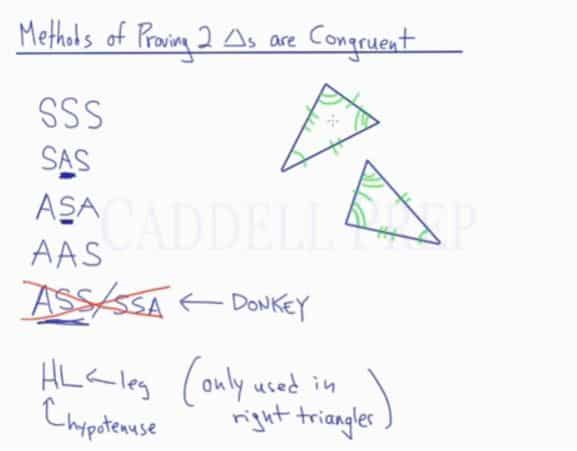In this video, you will learn the basics of proving triangles are congruent.

Congruence: Three sides of a triangle are congruent to their corresponding sides of another triangle. Two congruent triangles have the same angle measures and side lengths, so they have the same size as well.
Similarity: Three angles of a triangle are congruent to their corresponding angles of another triangle. Two similar triangles have the same angle measures but may differ in size.

Methods of Proving Two Triangles are Congruent:

• Side-Side-Side (SSS) Theorem – Three pairs of corresponding sides are congruent in two triangles
• Side-Angle-Side (SAS) Theorem – Two pairs of corresponding sides and the corresponding angle pair between the two sides are congruent in two triangles
• Angle-Side-Angle (ASA) Theorem – Two pairs of corresponding angles and the corresponding sides between those angles are congruent in two triangles
• Angle-Angle-Side (AAS) Theorem – Two pairs of corresponding angles and a pair of corresponding sides not between those angles are congruent in two triangles
• Hypotenuse-Leg (HL) Theorem – One pair of corresponding legs and the hypotenuses are congruent in two right triangles. *Be sure to prove that the triangles are right triangles first!

The Angle-Side-Side (ASS) or Side-Side-Angle (SSA) is not applicable to prove triangles congruent. They are commonly known as a donkey and are not acceptable. Use the HL Theorem instead.## Video-Lesson Transcript

In this lesson, we’ll go over methods of proving triangles are congruent.

What do congruent means?

Congruent means that all three angles of one triangle are the same as all three angles of the other triangle.

But not just same angles, because they are just similar, they should also have the same sides.

All the angles and all the sides should be congruent.

How do we prove that these two triangles are congruent with each other?

Well, we don’t have to prove each and every angle and side.

There are just a few things that we need to prove congruent. If those are true then we know for a fact that everything else is congruent as well.

Let’s go over that list.

Methods of proving triangles are congruent:

1. Side-Side-Side (SSS) – we have to prove that all three sides are congruent.

2. Side-Angle-Side (SAS) – what’s very important here is that the “Angle” is written between the two sides. Because in the diagram, the angle is in between two sides as well.

3. Angle-Side-Angle (ASA) – just like the “angle” in SAS is in between two sides; the “Side” here should also be in between two angles.

4. Angle-Angle-Side (AAS) – here, the “Side” is not in between two angles.

You should not use an Angle-Side-Side (ASS) method or Side-Side-Angle (SSA) method.

When you use any of these methods, you will be a donkey.

5. Hypotenuse-Leg (HL) – only used in right triangles.

Just a review, two triangles are congruent if everything about them is the same.

But to prove that they are congruent, we don’t have to individually prove each angle and side of these two triangles.

We just have to prove Side-Side-Side (SSS), Side-Angle-Side (SAS), Angle-Side-Angle (ASA), Angle-Angle-Side (AAS), or Hypotenuse-Leg (HL).

If we can prove any of these methods to be true, then we know that we have two congruent triangles.